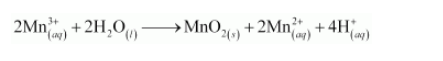# The Mn3+ ion is unstable in solution and undergoes disproportionation to give Mn2+,

Question:

The $\mathrm{Mn}^{3+}$ ion is unstable in solution and undergoes disproportionation to give $\mathrm{Mn}^{2+}, \mathrm{MnO}_{2}$, and $\mathrm{H}^{+}$ion. Write a balanced ionic equation for the reaction.

Solution:

The given reaction can be represented as: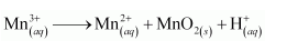The oxidation half equation is: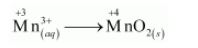The oxidation number is balanced by adding one electron as: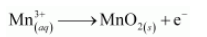The charge is balanced by adding 4H+ ions as: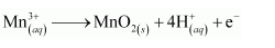The O atoms and H+ ions are balanced by adding 2H2O molecules as: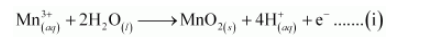The reduction half equation is: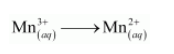The oxidation number is balanced by adding one electron as: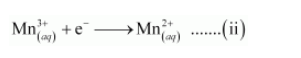The balanced chemical equation can be obtained by adding equation (i) and (ii) as: